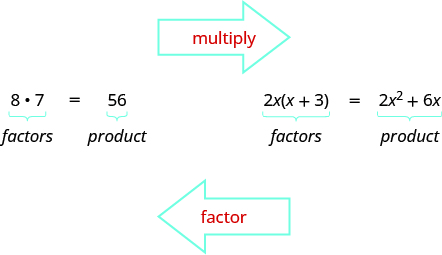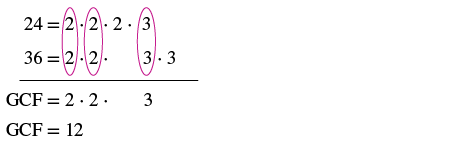Finding the Greatest Common Factor from Two Expressions

Learning Outcomes

• Find the greatest common factor of two numbers

Earlier we multiplied factors together to get a product. Now, we will be reversing this process; we will start with a product and then break it down into its factors. Splitting a product into factors is called factoring.We also factored numbers to find the least common multiple (LCM) of two or more numbers. Now we will factor expressions and find the greatest common factor of two or more expressions. The method we use is similar to what we used to find the LCM.

Greatest Common Factor

The greatest common factor (GCF) of two or more expressions is the largest expression that is a factor of all the expressions.

First we will find the greatest common factor of two numbers.

example

Find the greatest common factor of $24$ and $36$.

Solution

 Step 1: Factor each coefficient into primes. Write all variables with exponents in expanded form. Factor $24$ and $36$.Step 2: List all factors–matching common factors in a column.In each column, circle the common factors. Circle the $2, 2$, and $3$ that are shared by both numbers.Step 3: Bring down the common factors that all expressions share. Bring down the $2, 2, 3$ and then multiply. Step 4: Multiply the factors. The GCF of $24$ and $36$ is $12$.

Notice that since the GCF is a factor of both numbers, $24$ and $36$ can be written as multiples of $12$.

$\begin{array}{c}24=12\cdot 2\\ 36=12\cdot 3\end{array}$

try it

In the previous example, we found the greatest common factor of constants. The greatest common factor of an algebraic expression can contain variables raised to powers along with coefficients. We summarize the steps we use to find the greatest common factor.

Find the greatest common factor

1. Factor each coefficient into primes. Write all variables with exponents in expanded form.
2. List all factors—matching common factors in a column. In each column, circle the common factors.
3. Bring down the common factors that all expressions share.
4. Multiply the factors.

example

Find the greatest common factor of $5x\text{ and }15$.

try it

In the examples so far, the greatest common factor was a constant. In the next two examples we will get variables in the greatest common factor.

example

Find the greatest common factor of $12{x}^{2}$ and $18{x}^{3}$.

example

Find the greatest common factor of $14{x}^{3},8{x}^{2},10x$.

try it

Watch the following video to see another example of how to find the GCF of two monomials that have one variable.